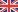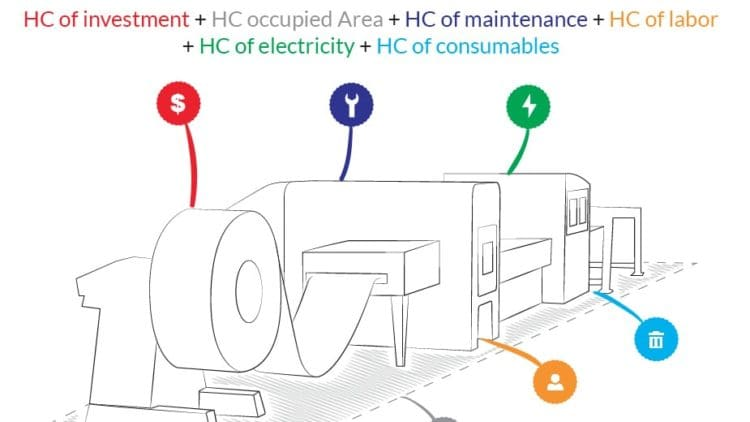Change language

# How to quickly calculate the hourly cost and rate of machines and sheet metal processing lines

February 2017I am often asked what is the operating cost for a sheet metal processing line, and in this article I will provide a method for calculating it.

We will see that, especially for new lines such as roll forming lines, punching machine and laser cutting, the payback and the number of working hours of the machine play the most significant role, and that the payback is a decision that the sheet metal manufacturer has to take.

Other times, my clients ask me how much they should charge for the machine per hour, and at the end of the article we will see that this question has a little different answer: in this case the entrepreneur has to decide how much of the overhead costs are connected to the machine operation, how much is the gross margin he wants to have and what is the hourly rate generally applied in the market.

You can find more details related to these concepts into the book “The Revolution of Efficiency” that you can request here:

## Calculating the hourly cost of a machine

The hourly cost of a machine is the sum of the following six factors, expressed in Hourly Cost (HC)

Let’s analyze each one of these factors.

1. Investment Hourly Cost

To calculate the Investment Hourly Cost, we start from the value of the investment and divide it by the number of years in which we want the machine to be paid back.

In accounting, the depreciation for a machine is generally 5 years but some companies want the payback to be completed within three years.

The obtained value has to be divided by the number of hours of expected operation of the line.

#### (Investment HC) = (Investment Value) / (Payback period) / (Estimated Hours of operation)

For example, an investment worth 500.000 Euro, with a Payback period of three years working 3000 hours per year will give a hourly cost of the investment of 55,5 Euro per hour.

If the machine is leased, alernatively it is possible to calculate the Investment HC by dividing the annual cost of the leasing by the number of hours of expected operation.

(Investment HC) = (Annual cost of Leasing) / (Estimated Hours of operation)

2. Electricity HC

Electricity Hourly Cost is calculated from the machine power consumption.

Power consumption is not the installed power that reads on the machine label, since that is a safety value that considers all machine utilities working simultaneously, which rarely happens. Power consumption can be measured by any electrician over a period of time with a specific instrument, and is sometimes 50% of the installed power (on servo electric punching lines, it can be even 15-20% of the installed power).

#### (Electricity HC) = (Machine power consumption in kW) * (Cost of electricity in [Euro/kWh])

For example, a machine with power consumption of 20kW gives an Electricity HC of about 3 Euro per working hour.

New servo electric technologies are reducing considerably the power consumption: for example one modern servo electric coil fed punching machine (see picture below) has a power consumption of less than 12kW, compared to over 30kW of an hydraulic punching machine with similar characteristics.

3. Labor HC

This is the labor cost that is directly involved to assist the machine. In some cases one operator can assist more than one machine, and this cost has to take into account the percentage of his/her time per each machine.

#### (Labor HC) = (Operator HC) * (% of time for machine assistance)

For example, if an operator has a cost of 25 Euro per hour and assists the machine 35% of its time, the Labor HC is 8,8 Euro per hour. In more automated machines, the percentage is lower than for machines with low or no automation.

4. Maintenance HC

To calculate this cost, we can divide the maintenance yearly costs by the number of estimated operating hours of the machine. The cost of the maintenance can be derived from the maintenance costs of similar machines in the workshop, or it can be estimated as a percentage of the investment value.

#### (Maintenance HC) = (Maintenance Yearly cost) / (Estimated Hours of operation)

For example, a machine with 6.000 Euro Yearly maintenance costs with 3000 Hours of estimated operating hours, has a Maintenance HC of 2 Euro per hour.

5. Consumables HC

Consumables are, for example, the cost of wear parts such as punches and dies, filters, lubricants, or the assist gas for laser cutting machines or lines that include welding.

These costs can be derived from historical values of similar machines, or calculated and they are a direct function of the number of operating hours of the line.

In the following example, we will consider a Consumables HC of 8 Euro.

6. Occupied Area Hourly Cost

For sake of completeness, we can add the hourly cost of the area occupied by the machine. I suggest considering the yearly cost for renting a similar area and divide it by the number of Estimated Hours of operation in the year.

#### (Occupied Area HC) = (Cost of rented area per year) / ( Estimated Hours of operation)

In the following example, we will consider this cost as zero.

### Total Hourly Cost

In the previous example, the Total Hourly Cost results in 77,3 Euro. This cost covers the machine or line operating costs and as we have seen it already involved the decision on the payback period.

The Investment HC for new lines is usually the most important factor of the sum. For machines that have completed their payback or depreciation period, this factors can be considered zero; usually a higher value has to be calculated for the Maintenance HC.

Notes

When calculating the cost of a production for a customer, the HC is used in this formula:

#### (Total production cost) = (number of parts) * (raw material cost per one part) + (HC) * [(Cycle time per part in hours) * (number of parts) + (Setup time in hours)] + (cost of tooling) + (cost of machine programming)

We observe the following:

The Hourly cost multiplied by the number of parts and cycle time, is a variable cost that depends on the total number of produced parts.

The time for the setup of the machine can be multiplied by HC as well, since the machine is standing in this time. This can be considered a fixed cost. As we will see in one of the next articles, it is possible to consider the setup time by using the parameter Efficiency for the system.

If the production requires the manufacturing of a tooling that is production-specific, this is also considered a fixed cost, just like the cost of the machine programming.

## Deciding the hourly price for the sheet metal working machine

The previous calculations give us a framework for the pricing of our machine, per hour of operation.

Knowing the machine hourly cost, the entrepreneur has now to add two more factors: the repartition of the overhead costs, and the gross margin he wants to get from the machine operation.

Overhead costs are the company structural costs such as commercial costs, investments, maintenance, heating, administration and service costs that are not directly connected with the production. There is not a fixed rule for the repartition of these costs, but I suggest this formula:

#### (Overhead cost repartition HC) = (Overhead costs) / (Total production area in square meters) * (Machine occupied area in square meters) / (Estimated Hours of operation)

In this way, a machine occupying a smaller surface on the shop floor will “absorb” less overhead costs than the larger machines.

Note: in the Machine Hourly cost, we already included the hourly cost of the investment of the machine – either as leasing cost or depreciation – and the power consumption of the machine. These costs are generally included in the Overhead costs: for the sake of accuracy, I suggest to deduct the yearly cost of the Investment and the yearly estimated power consumption of the machine from the (Overhead costs) in the above formula. By doing so, we obtain a less conservative calculation.

### Margin

We know that the cost is a calculation, while price is a decision. In fact, knowing the operating costs is an essential step to a correct pricing, and also for the evaluation of a new investment.

To calculate the price, we now need to add the margin that we want to have on the worked hour, in percentage. Here is the formula:

#### (Hourly price) = ((Machine HC) + (Overhead cost repartition HC)) / (100 – Margin%) * 100

For example, we had found that our system had a HC of 77,3 Euros per hour. If the Overhead cost repartition is 12 Euro per hour and we want to have a margin of 15%, the final hourly price results in:

(Hourly price) = (77,3 + 12) / (100-15) * 100 = 105 Euro per Hour.

The sheet metal manufacturer should also be informed on the pricing that is generally applied in the market for the same machine type, and consider this in his final decision.

## Conclusions

In this article I have shared a simple method for the calculation of the machine hourly cost, which can be valid for a number of sheet metal working machines and applied even outside the sheet metal industry.

All the calculations imply some evaluations and strategic decisions that the sheet metal manufacturer has to take, so it is quite common to see two companies applying different hourly prices, based on their different policies on payback time or structural cost repartition.

In any case, in order to take a thoughtful and effective decision on the pricing, the entrepreneur has to be aware also of the price per hour applied in the market by other manufacturers in his area.

Author
Andrea Dallan – C.E.O.